Anúncio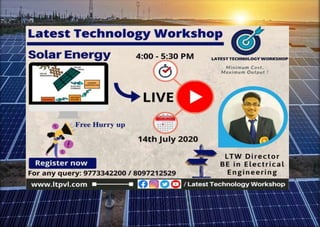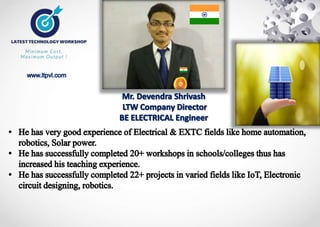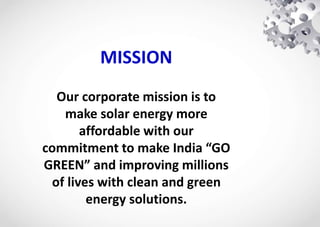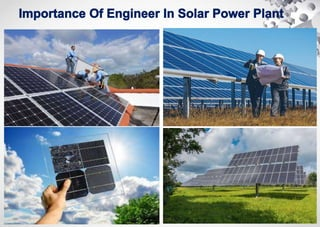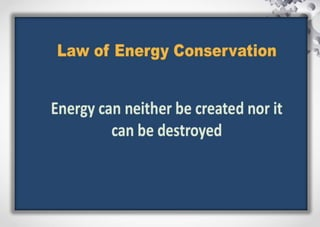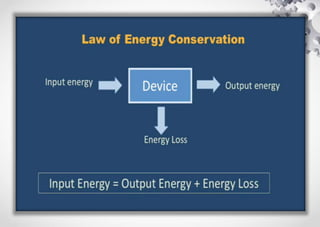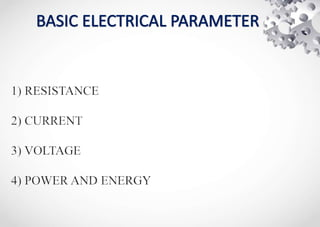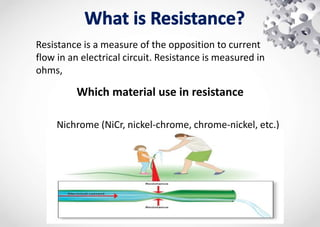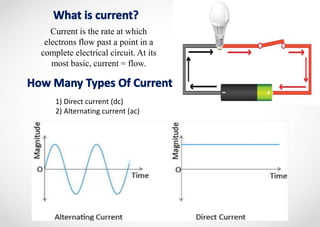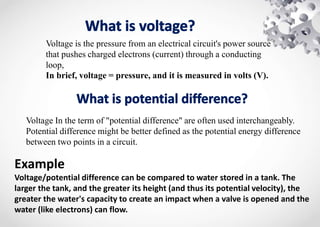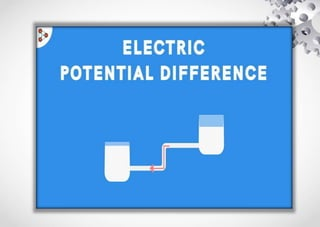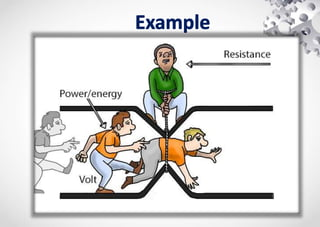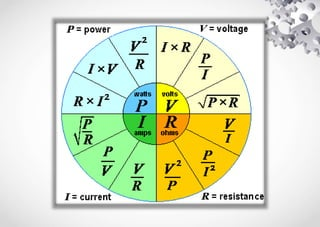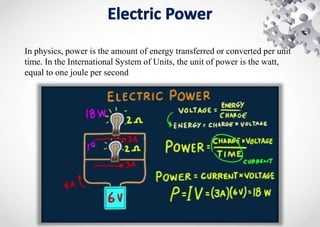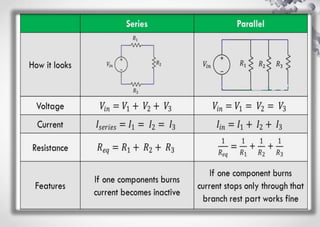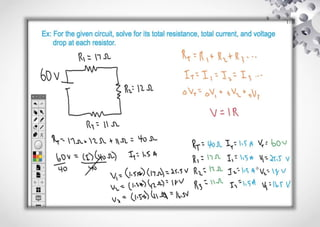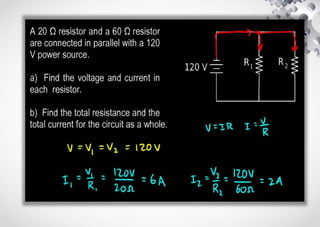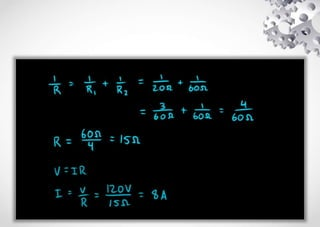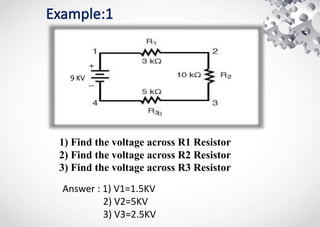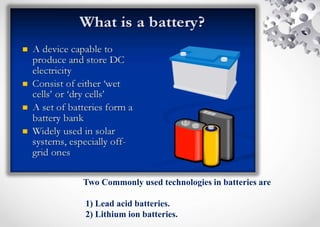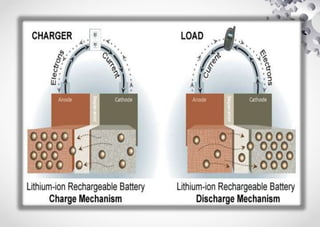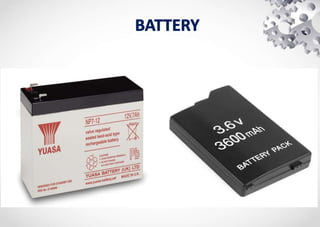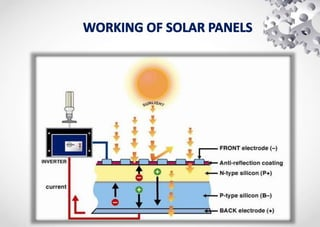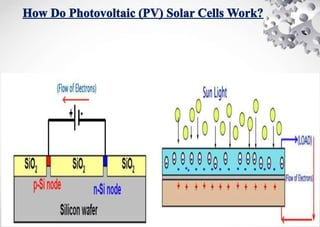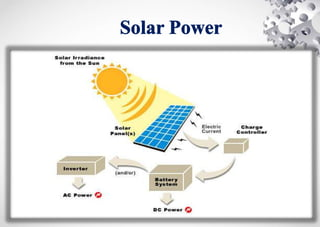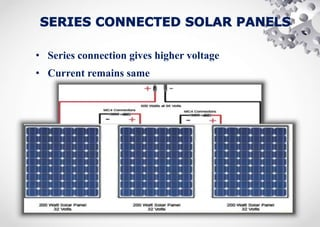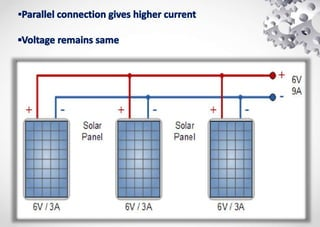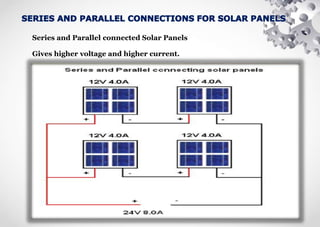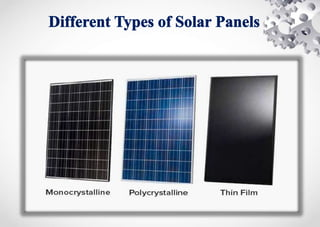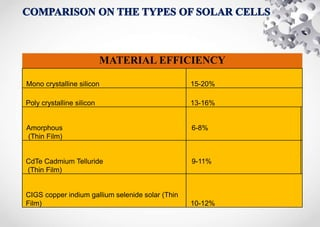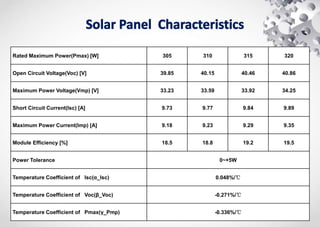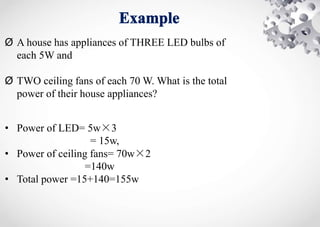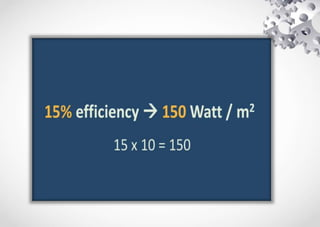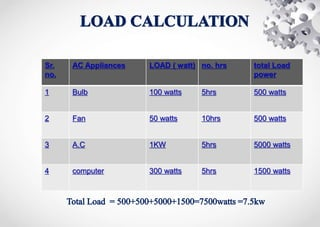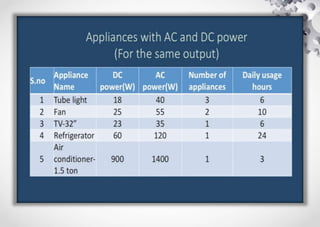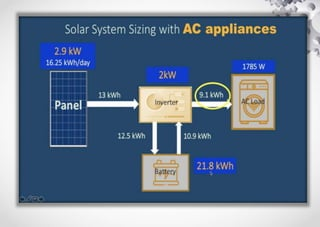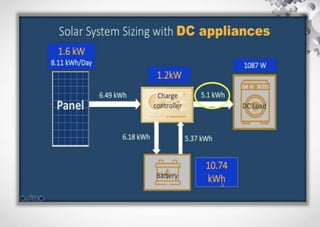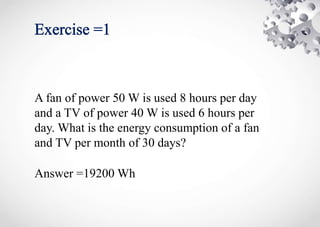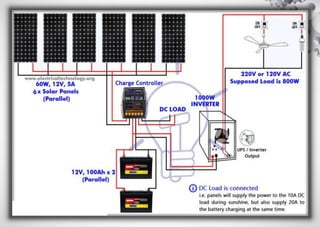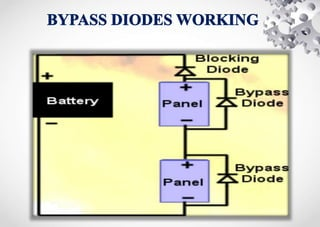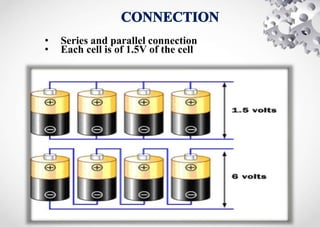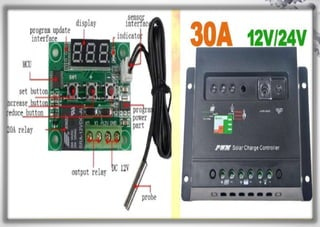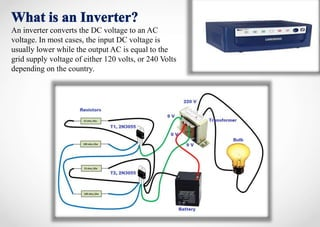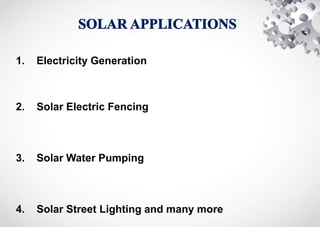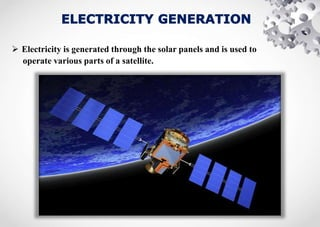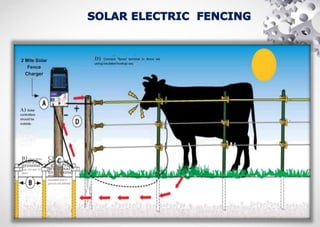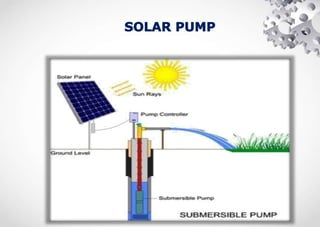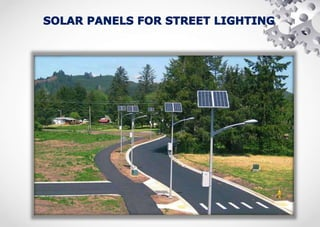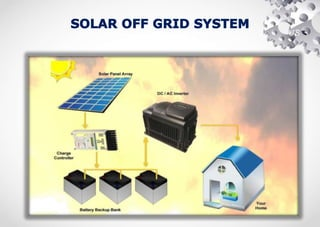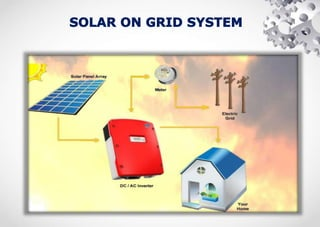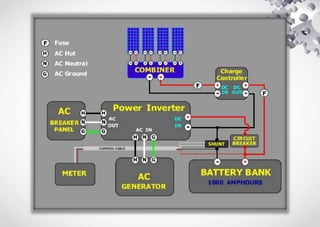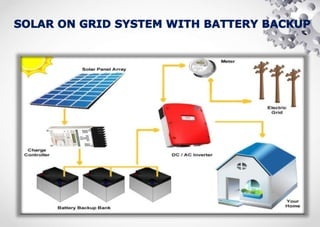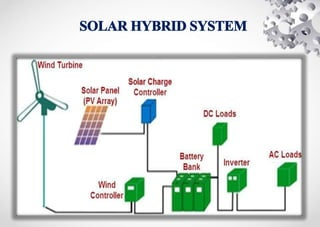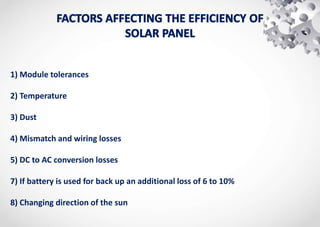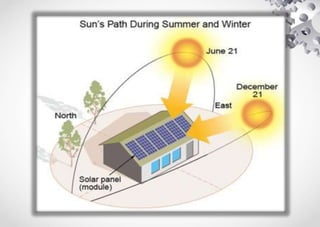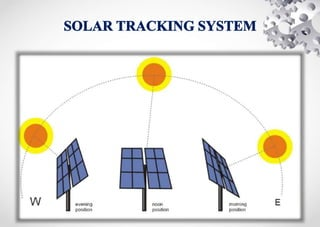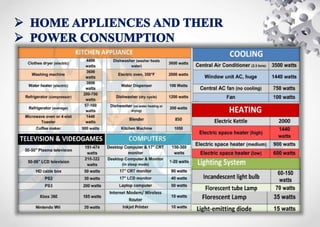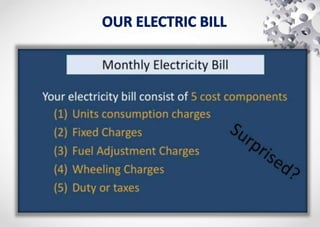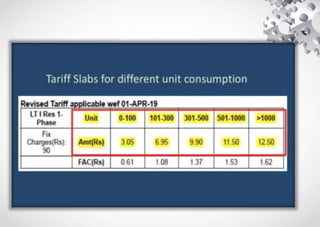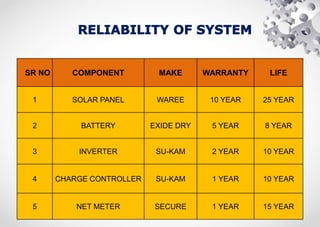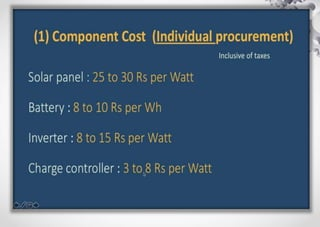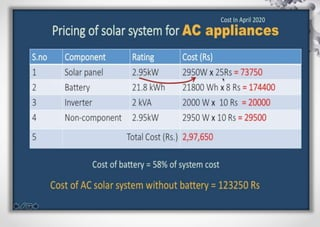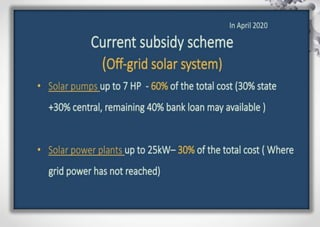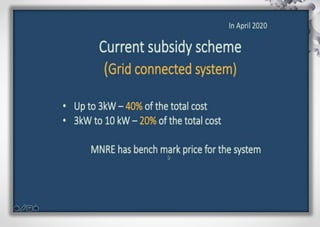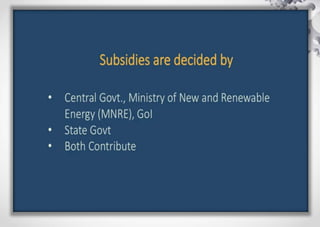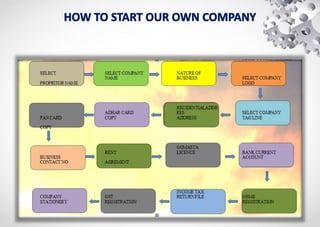1 de 70
Anúncio

### DEVENDRA SHRIVASH SOLAR POWER.ppt

1. MISSION Our corporate mission is to make solar energy more affordable with our commitment to make India “GO GREEN” and improving millions of lives with clean and green energy solutions.
2. Which material use in resistance Nichrome (NiCr, nickel-chrome, chrome-nickel, etc.) Resistance is a measure of the opposition to current flow in an electrical circuit. Resistance is measured in ohms,
3. 1) Direct current (dc) 2) Alternating current (ac) Current is the rate at which electrons flow past a point in a complete electrical circuit. At its most basic, current = flow.
4. Example Voltage/potential difference can be compared to water stored in a tank. The larger the tank, and the greater its height (and thus its potential velocity), the greater the water's capacity to create an impact when a valve is opened and the water (like electrons) can flow. Voltage is the pressure from an electrical circuit's power source that pushes charged electrons (current) through a conducting loop, In brief, voltage = pressure, and it is measured in volts (V). Voltage In the term of "potential difference" are often used interchangeably. Potential difference might be better defined as the potential energy difference between two points in a circuit.
5. In physics, power is the amount of energy transferred or converted per unit time. In the International System of Units, the unit of power is the watt, equal to one joule per second
6. 1) Find the voltage across R1 Resistor 2) Find the voltage across R2 Resistor 3) Find the voltage across R3 Resistor Answer : 1) V1=1.5KV 2) V2=5KV 3) V3=2.5KV  9 KV
7. k 1) Find the current across R1 Resisto 2) Find the current across R2 Resistor 3) Find the current across R3 Resistor ANSWER : I1=9÷10=0.9KA I2=9÷2=4.5KA I3= 9÷1=9KA TOTAL CURRENT= 14.4KA TOTAL RERSISTANCE=0.625 I=9÷0.625= 14.4KA 9 KV
8. Two Commonly used technologies in batteries are 1) Lead acid batteries. 2) Lithium ion batteries.
9. • Series connection gives higher voltage • Current remains same
10. Series and Parallel connected Solar Panels Gives higher voltage and higher current.
11. MATERIAL EFFICIENCY Mono crystalline silicon 15-20% Poly crystalline silicon 13-16% Amorphous (Thin Film) 6-8% CdTe Cadmium Telluride (Thin Film) 9-11% CIGS copper indium gallium selenide solar (Thin Film) 10-12%
12. Rated Maximum Power(Pmax) [W] 305 310 315 320 Open Circuit Voltage(Voc) [V] 39.85 40.15 40.46 40.86 Maximum Power Voltage(Vmp) [V] 33.23 33.59 33.92 34.25 Short Circuit Current(Isc) [A] 9.73 9.77 9.84 9.89 Maximum Power Current(Imp) [A] 9.18 9.23 9.29 9.35 Module Efficiency [%] 18.5 18.8 19.2 19.5 Power Tolerance 0~+5W Temperature Coefficient of Isc(α_Isc) 0.048%/℃ Temperature Coefficient of Voc(β_Voc) -0.271%/℃ Temperature Coefficient of Pmax(γ_Pmp) -0.336%/℃
13. Ø A house has appliances of THREE LED bulbs of each 5W and Ø TWO ceiling fans of each 70 W. What is the total power of their house appliances? • Power of LED= 5w×3 = 15w, • Power of ceiling fans= 70w×2 =140w • Total power =15+140=155w
14. Sr. no. AC Appliances LOAD ( watt) no. hrs total Load power 1 Bulb 100 watts 5hrs 500 watts 2 Fan 50 watts 10hrs 500 watts 3 A.C 1KW 5hrs 5000 watts 4 computer 300 watts 5hrs 1500 watts
15. A fan of power 50 W is used 8 hours per day and a TV of power 40 W is used 6 hours per day. What is the energy consumption of a fan and TV per month of 30 days? Answer =19200 Wh
16. • Series and parallel connection • Each cell is of 1.5V of the cell
17. An inverter converts the DC voltage to an AC voltage. In most cases, the input DC voltage is usually lower while the output AC is equal to the grid supply voltage of either 120 volts, or 240 Volts depending on the country.
18. 1. Electricity Generation 2. Solar Electric Fencing 3. Solar Water Pumping 4. Solar Street Lighting and many more
19.  Electricity is generated through the solar panels and is used to operate various parts of a satellite.
20. D) Connect "fence' terminal to fence we usinginsulatedhookup wire. 2 Mile Solar Fence Charger A) Solar controllers should be outside. C) Connect "grocrrd* terminal to ground rods with insulated wire to ground rod damps. B) Drive three 6‘orJT ground rods vito sod 10’ apart.
21. US
22. 1) Module tolerances 2) Temperature 3) Dust 4) Mismatch and wiring losses 5) DC to AC conversion losses 7) If battery is used for back up an additional loss of 6 to 10% 8) Changing direction of the sun
23. SR NO COMPONENT MAKE WARRANTY LIFE 1 SOLAR PANEL WAREE 10 YEAR 25 YEAR 2 BATTERY EXIDE DRY 5 YEAR 8 YEAR 3 INVERTER SU-KAM 2 YEAR 10 YEAR 4 CHARGE CONTROLLER SU-KAM 1 YEAR 10 YEAR 5 NET METER SECURE 1 YEAR 15 YEAR
Anúncio